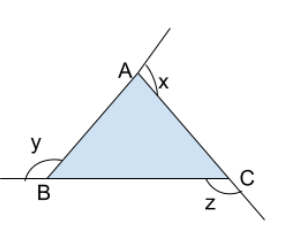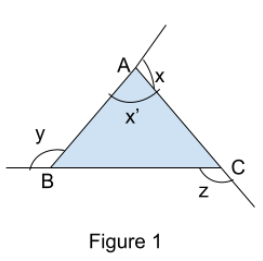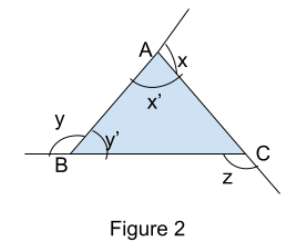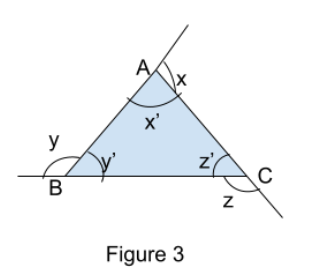Courses
Courses for Kids
Free study material
Offline Centres
MoreLast updated date: 06th Dec 2023
Total views: 281.4k
Views today: 3.81k

# In $\Delta ABC$ fig. $\angle x+\angle y+\angle z$ is equals to:A. 120B. 180C. 240D. 360Verified
281.4k+ views
Hint: We can solve these triangle angle problems easily, through the angle sum property of triangle and linear angle sum property. Which states that the sum of all the angle of triangle is ${{180}^{{}^\circ }}$ and the linear sum angle of line is also ${{180}^{{}^\circ }}$ . Here in this question as we know that sum of all the angles of triangle is ${{180}^{{}^\circ }}$ and also that the sum of interior and exterior angle on a straight line is also ${{180}^{{}^\circ }}$ (linear angle sum property), so we can write the exterior angle in terms of interior angle of triangle and then sum up all the exterior angle (as asked in question) whose sum we know through the angle sum property of triangle. So we will get the answer.To understand it in more detail let us solve the question; $,$ are the straight lines through which a triangle is formed. As in the figure the exterior angles are marked at $\angle x,\angle y,\angle z$ . As we know that in a line sum of angle is equal to ${{180}^{{}^\circ }}$ i.e. in figure 1, AB is a line, on which angle $\angle x,\angle x'$ are present. So by linear angle sum property we can say that $\angle x+\angle x'={{180}^{{}^\circ }}$
Similarly, with the line sum of angle is equal to ${{180}^{{}^\circ }}$ i.e. in figure 2,BC is a line , on which angle $\angle y,\angle y'$ are present. So by linear angle sum property we can say that $\angle y+\angle y'={{180}^{{}^\circ }}$ in which $\angle y$ is the exterior angle and $\angle y'$ is the interior angle of triangle.
Similarly, the line sum of angle is equal to ${{180}^{{}^\circ }}$ i.e. in figure 3 on line $AC$ , on which angle $\angle z,\angle z'$ are present. So by linear angle sum property we can say that $\angle z+\angle z'={{180}^{{}^\circ }}$ in which $\angle z$ is the exterior angle and $\angle z'$ is the interior angle of triangle.Now in $\vartriangle ABC$ whose interior angles are $\angle x',\angle y',\angle z'$ and exterior angle are equal to $\angle x,\angle y,\angle z$ . As we know, the sum of all interior angles is ${{180}^{{}^\circ }}$ by angle sum property of triangle. So we can write $\angle x'+\angle y'+\angle z'={{180}^{{}^\circ }}$
So from above explanation we have
$\angle x+\angle x'={{180}^{{}^\circ }}$ equation (i)
$\angle y+\angle y'={{180}^{{}^\circ }}$ equation (ii)
$\angle z+\angle z'={{180}^{{}^\circ }}$ equation (iii)
$\angle x'+\angle y'+\angle z'={{180}^{{}^\circ }}$ equation (iv)
Adding equation (i), (ii), (iii), i.e.
\begin{align} & \left( i \right)+\left( ii \right)+\left( iii \right) \\ & \angle x+\angle x'+\angle y+\angle y'+\angle z+\angle z'={{180}^{{}^\circ }}+{{180}^{{}^\circ }}+{{180}^{{}^\circ }} \\ & \angle x+\angle x+\angle z+\angle x'+\angle y'+\angle z'=540 \\ \end{align}
Now we can replace; $\angle x'+\angle y'+\angle z'$with ${{180}^{{}^\circ }}$ from equation (iv), so we will get
\begin{align} & \angle x+\angle x+\angle z+\angle x'+\angle y'+\angle z'=540 \\ & \angle x+\angle x+\angle z+{{180}^{{}^\circ }}={{540}^{{}^\circ }} \\ & \angle x+\angle x+\angle z={{360}^{{}^\circ }} \\ \end{align}
Hence answer is${{360}^{{}^\circ }}$, i.e. $\angle x+\angle y+\angle z={{360}^{{}^\circ }}$

So, the correct answer is “Option D”.

Note: To find the angle or sum of angles in a triangle, then angle sum property is the basic you must know, then linear pair of angle or linear angle sum property. Moreover the sum of exterior angle is also called as two times the sum of interior angle of triangle, as it is${{360}^{{}^\circ }}$which is twice of ${{180}^{{}^\circ }}$